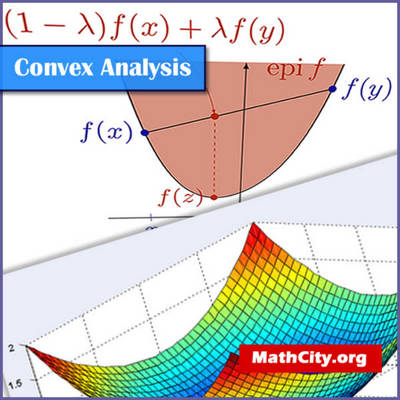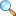# MTH424: Convex Analysis (Fall 2020)At the end of this course the students will be able to understand the concept of Convex Analysis, convex sets, convex functions, Differential of the convex function. Developing ability to study the Hadamard-Hermite inequalities and their applications. Prepare students to be self independent and enhance their mathematical ability by giving them home work and projects.

Convex sets and their properties, Convex hull and their properties, Best approximation theorem. Convex functions, Basic definitions, properties, various generalizations, Differentiable convex functions, Hermite and Hadamard inequalities, Subgradient, Characterizations and applications in linear and nonlinear optimization.

The main aim of this course is to learn about convex functions and discuss it properties. Here we give objective & sample questions lecture wise. All the recorded lectures are given on Microsoft Team.

#### Lecture 01

• Definitions: Interval, convex function, strictly convex function, concave function, strictly concave function
• Example of convex & concave functions
• By definition, prove that $f(x)=x$ is convex on $\mathbb{R}$.
• By definition, prove that $f(x)=x^2$ is convex on $\mathbb{R}$.

#### Lecture 02

• Prove that every convex function on closed interval is bounded.
• Prove that every convex function on closed interval is bounded below.
• Prove that every convex function on closed interval is bounded above.
• If $f:[a,b]\to \mathbb{R}$ is convex, then $f$ is bounded above by $\max(f(a),f(b))$.

#### Lecture 03

• Definitions: Continuity, uniform continuity.
• Prove that every convex function is continuous on its interior of its domain.

#### Lecture 04

• Left & right derivative
• If $f:I\rightarrow \mathbb{R}$ is convex, then $f_{-}'(x)$ and $f_{+}'(x)$ exist and are increasing on $I^{\circ}$

#### Lecture 05

• A function $f:(a,b)\rightarrow \mathbb{R}$ is convex if and only if there is increasing function $g:(a,b)\rightarrow \mathbb{R}$ and a point $c\in (a,b)$ such that for all $x\in (a,b)$,

$$f(x)-f(c)=\int^{x}_{c} g(t)dt.$$

• Suppose $f$ is differentiable on $(a,b)$. Then $f$ is convex [strictly convex] if, and only if, $f'$ is increasing [strictly increasing] on $(a,b)$.
• Prove that $x^2$ is convex on $(-\infty, \infty)$.
• Prove that $-\log x$ is convex on $(0,\infty)$.
• Prove that $x^3$ is convex on $(0,\infty)$.
• Prove that $x^4$ is convex on $(-\infty,\infty)$.

#### Lecture 06

• Let $f$ is twice differentiable on $(a,b)$. Then $f$ is convex on $(a,b)$ iff $f''(x)\geq 0$ for all $x\in(a,b)$. If $f''(x)>0$ for all $x\in(a,b)$, then $f$ is strictly convex on $(a,b)$.
• If $f:I\rightarrow \mathbb{R}$ and $g:I\rightarrow \mathbb{R}$ are convex then $f+g$ is convex on $I$.
• If $f:I\rightarrow \mathbb{R}$ is convex

and $\alpha \geq 0$, then $\alpha f$ is convex on $I$.

#### Lecture 07

• Definition: Line of support
• A function $f: (a, b)\to \mathbb{R}$ is convex if and only if there is at least one line of support for $f$ at each $x_0\in (a, b)$.
• Find the line of support for $\exp (x)$ at $x=1$.

#### Lecture 08

• Find the line of supports for the function defined below at $x=1$.

$$f(x)=\begin{cases} x^2, \quad x\geq 1; \\ x, \quad x<1. \end{cases}$$

• Find the line of support for $f(x)=x^2$ at $x=2$.

#### Lecture 09

• Let $f:I\rightarrow \mathbb{R}$ and $g:J\rightarrow \mathbb{R}$ where $range\left( f\right) \subseteq J$. If $f\$and$\ g$ are convex and $g$ is increasing, then the composite function $g\circ f\$ is convex on $I$.
• Prove that $h(x)=|x+5|^p$, where $p>1$ is convex on $(-\infty,\infty)$.

#### Lecture 10

• Let $f_{\alpha}:I\to \mathbb{R}$ be an arbitrary family of convex functions and let $f(x)=\sup_{\alpha}f_{\alpha}(x)$. If $J=[x \in I : f(x) < \infty]$ is non empty then $J$ is an interval and $f$ is convex on $J$.
• If $f_n:I\to \mathbb{R}$ is a sequence of convex functions converging to a finite limit function $f$ on $I$, then $f$ is convex on $I$.

#### Lecture 11

• If $f:I\rightarrow \mathbb{R}$ and $g:I\rightarrow \mathbb{R}$ are both non-negative, decreasing and convex functions, then $h(x)=f(x)g(x)$ is also non-negative, decreasing and convex on $I$.
• Quiz 01 | Download PDF |View Online
• Hint: If $f$ is differentiable and increasing on $[a,b]$, then $f'(x)\geq 0$ for $x\in [a,b]$.
• Quiz/Assignment 04 | Download PDF |View OnlinePlease click on View Online to see inside the PDF.

1. A. W. Roberts and D. E. Varberg, Convex Functions, Academic Press, New York, 1973. (Google Book Preview)
2. Nonlinear Programming Theory and Algorithms, 3rd Edition, by M. S. Bazaraa, H. D. Sherali and C. M. Shetty.
3. Convex Functions and Their Applications, A Contemporary Approach, by C. P. Niculescu and L. E. Persson.
4. Convex Analysis and Monotone Operator Theory in Hilbert Spaces, by H. H. Bauschke and P. L. Combettes.
• atiq/fa20-mth424